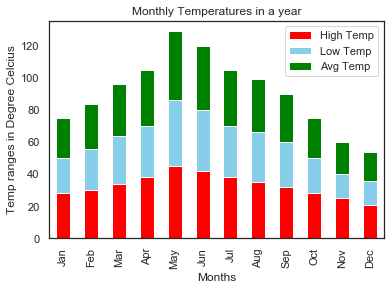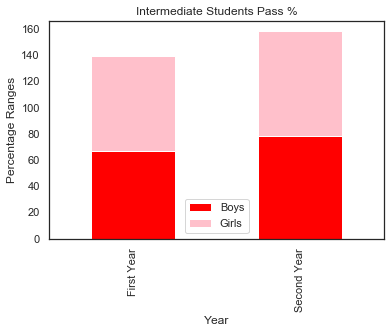# How to Create a Stacked Bar Plot in Seaborn?

• Last Updated : 18 Feb, 2022

In this article, we will discuss how to create stacked bar plot in Seaborn in Python.

A stacked Bar plot is a kind of bar graph in which each bar is visually divided into sub bars to represent multiple column data at once. To plot the Stacked Bar plot we need to specify stacked=True in the plot method. We can also pass the list of colors as we needed to color each sub bar in a bar.

Syntax:

DataFrameName.plot( kind=’bar’, stacked=True, color=[…..])

Example: Stacked bar plot

Dataset in use:

## Python3

 `# import necessary libraries``import` `pandas as pd``import` `seaborn as sns``import` `matplotlib.pyplot as plt` `# create DataFrame``df ``=` `pd.DataFrame({``'High Temp'``: [``28``, ``30``, ``34``, ``38``, ``45``, ``42``,``                                 ``38``, ``35``, ``32``, ``28``, ``25``, ``21``],``                   ``'Low Temp'``: [``22``, ``26``, ``30``, ``32``, ``41``, ``38``,``                                ``32``, ``31``, ``28``, ``22``, ``15``, ``15``],``                   ``'Avg Temp'``: [``25``, ``28``, ``32``, ``35``, ``43``, ``40``,``                                ``35``, ``33``, ``30``, ``25``, ``20``, ``18``]},``                  ``index``=``[``'Jan'``, ``'Feb'``, ``'Mar'``, ``'Apr'``, ``'May'``,``                         ``'Jun'``, ``'Jul'``, ``'Aug'``, ``'Sep'``, ``'Oct'``,``                         ``'Nov'``, ``'Dec'``])`  `# create stacked bar chart for monthly temperatures``df.plot(kind``=``'bar'``, stacked``=``True``, color``=``[``'red'``, ``'skyblue'``, ``'green'``])` `# labels for x & y axis``plt.xlabel(``'Months'``)``plt.ylabel(``'Temp ranges in Degree Celsius'``)` `# title of plot``plt.title(``'Monthly Temperatures in a year'``)`

Output:Example: Stacked bar plot

Dataset in use:

## Python3

 `# import necessary libraries``import` `pandas as pd``import` `seaborn as sns``import` `matplotlib.pyplot as plt` `# create DataFrame``students ``=` `pd.DataFrame({``'Boys'``: [``67``, ``78``],``                         ``'Girls'``: [``72``, ``80``], },``                        ``index``=``[``'First Year'``, ``'Second Year'``])`  `# create stacked bar chart for students DataFrame``students.plot(kind``=``'bar'``, stacked``=``True``, color``=``[``'red'``, ``'pink'``])` `# Add Title and Labels``plt.title(``'Intermediate Students Pass %'``)``plt.xlabel(``'Year'``)``plt.ylabel(``'Percentage Ranges'``)`

OutputMy Personal Notes arrow_drop_up